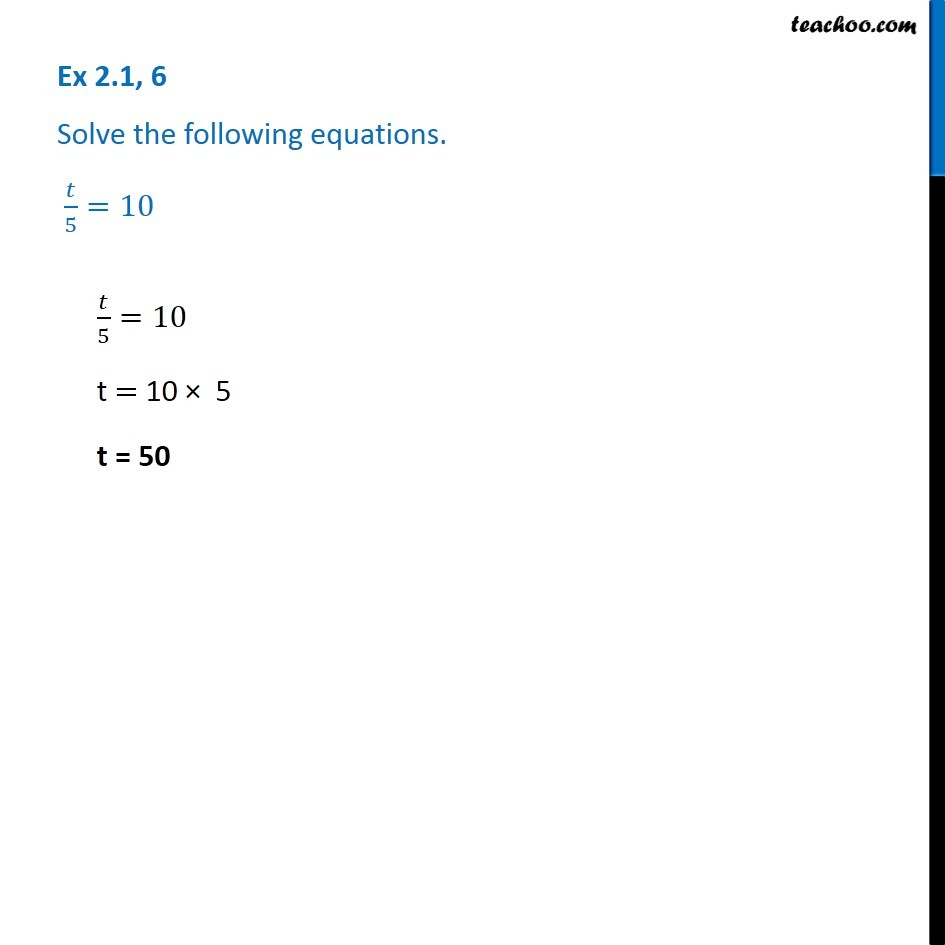Solving easy equations

Chapter 2 Class 8 Linear Equations in One Variable
Serial order wiseLearn in your speed, with individual attention - Teachoo Maths 1-on-1 Class

### Transcript

Question 6 - Chapter 2 Class 8 NCERT - Linear Equations in one variable Solve the following equations. t/5=10 t/5=10 Taking 5 to the right side t = 10 × 5 t = 50